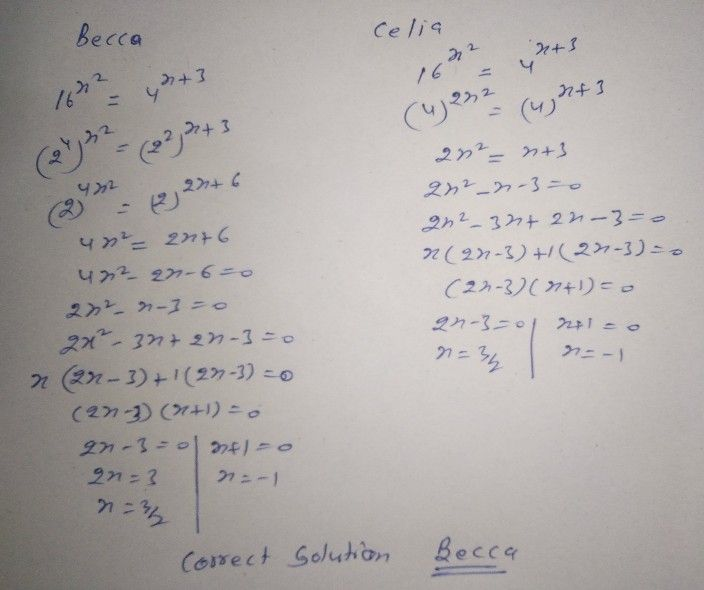Symbol
ProblemEngagement HIuitgeh g Solving Exponential Equations and Inequalities Purp- I. Find the Missing x! given $05recti0n$ Solve for the values $of\times lo5$ each ot the following exponential equations and inequalities. $82-x=2$ 1.82-x 2. $\left(1/2\right)x<1/8$ $5x=25x-2$ 3.5 4.3 $3x+2\geq 27$ 5.43 $43x=8x-1$ II. Who Has a Point? $0irecton$ Observe each of the following pairs of solutions. Decide whether anyone of them got the $corFec$ answer. $Answe$ the sets of guide questions. Becca Celia 16T 4*+3 $16x^{2}=4^{x+3}$ $\left(4\right)^{2x^{2}}=4x+$ $\left(2\right)^{4x^{2}}=\left(2\right)^{2\left(x+3\right)}$ $4x^{2}=2x+6$ $2x^{2}=x+3$ $2x^{2}-x-3$ G) $\left(4x^{2}-2x-6=0\right)$ 0 $2x^{2}-x-3=0$ $\left(2x-3\right)\left(x+1\right)=0$ $2x-3=0andx+1=0$ $\left(2x-3\right)\left(x+1\right)=0$ $2x-3=0andx+1=0$ $x=\dfrac {3} {2}x=-1$ $x=\dfrac {3} {2},x=-1$ 1. How did you check whether 3/2 and -1 are really solutions of the given exponential equation? 2. Were Becca and Celia both correct or both wrong?
10th-13th grade
Other
Search count: 112
SolutionQanda teacher - LokeshStudent
how to arrange??